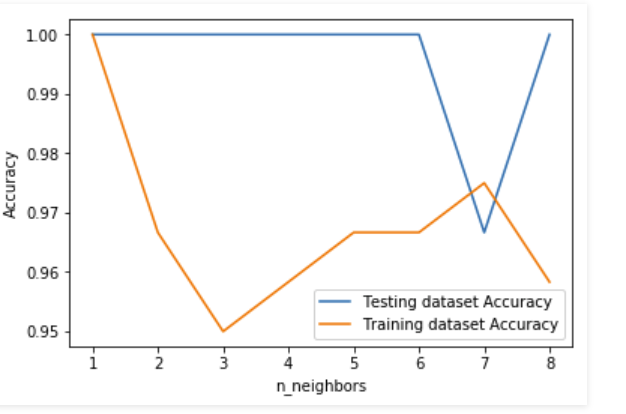# k-nearest neighbor algorithm in Python

Supervised Learning :
It is the learning where the value or result that we want to predict is within the training data (labeled data) and the value which is in data that we want to study is known as Target or Dependent Variable or Response Variable.
All the other columns in the dataset are known as the Feature or Predictor Variable or Independent Variable.

Supervised Learning is classified into two categories:

1. Clarification: Here our target variable consists of the categories.
2. Regression: Here our target variable is continuous and we usually try to find out the line of the curve.

As we have understood that to carry out supervised learning we need labeled data. How we can get labeled data? There are various ways to get labeled data:

1. Historical labeled Data
2. Experiment to get data: We can perform experiments to generate labeled data like A/B Testing.
3. Crowd-sourcing

Now it’s time to understand algorithms that can be used to solve supervised machine learning problem. In this post, we will be using popular `scikit-learn` package.

Note: There are few other packages as well like TensorFlow, Keras etc to perform supervised learning.

## k-nearest neighbor algorithm:

This algorithm is used to solve the classification model problems. K-nearest neighbor or K-NN algorithm basically creates an imaginary boundary to classify the data. When new data points come in, the algorithm will try to predict that to the nearest of the boundary line.

Therefore, larger k value means smother curves of separation resulting in less complex models. Whereas, smaller k value tends to overfit the data and resulting in complex models.

Note: It’s very important to have the right k-value when analyzing the dataset to avoid overfitting and underfitting of the dataset.

Using the k-nearest neighbor algorithm we fit the historical data (or train the model) and predict the future.

#### Example of the k-nearest neighbor algorithm

 `# Import necessary modules ` `from` `sklearn.neighbors ``import` `KNeighborsClassifier ` `from` `sklearn.model_selection ``import` `train_test_split ` `from` `sklearn.datasets ``import` `load_iris ` ` `  `# Loading data ` `irisData ``=` `load_iris() ` ` `  `# Create feature and target arrays ` `X ``=` `irisData.data ` `y ``=` `irisData.target ` ` `  `# Split into training and test set ` `X_train, X_test, y_train, y_test ``=` `train_test_split( ` `             ``X, y, test_size ``=` `0.2``, random_state``=``42``) ` ` `  `knn ``=` `KNeighborsClassifier(n_neighbors``=``7``) ` ` `  `knn.fit(X_train, y_train) ` ` `  `# Predict on dataset which model has not seen before ` `print``(knn.predict(X_test)) `

In the example shown above following steps are performed:

1. The k-nearest neighbor algorithm is imported from the scikit-learn package.
2. Create feature and target variables.
3. Split data into training and test data.
4. Generate a k-NN model using neighbors value.
5. Train or fit the data into the model.
6. Predict the future.

We have seen how we can use K-NN algorithm to solve the supervised machine learning problem. But how to measure the accuracy of the model?

Consider an example shown below where we predicted the performance of the above model:

 `# Import necessary modules ` `from` `sklearn.neighbors ``import` `KNeighborsClassifier ` `from` `sklearn.model_selection ``import` `train_test_split ` `from` `sklearn.datasets ``import` `load_iris ` ` `  `# Loading data ` `irisData ``=` `load_iris() ` ` `  `# Create feature and target arrays ` `X ``=` `irisData.data ` `y ``=` `irisData.target ` ` `  `# Split into training and test set ` `X_train, X_test, y_train, y_test ``=` `train_test_split( ` `             ``X, y, test_size ``=` `0.2``, random_state``=``42``) ` ` `  `knn ``=` `KNeighborsClassifier(n_neighbors``=``7``) ` ` `  `knn.fit(X_train, y_train) ` ` `  `# Calculate the accuracy of the model ` `print``(knn.score(X_test, y_test)) `

Model Accuracy:
So far so good. But how to decide the right k-value for the dataset? Obviously, we need to be familiar to data to get the range of expected k-value, but to get the exact k-value we need to test the model for each and every expected k-value. Refer to the example shown below.

 `# Import necessary modules ` `from` `sklearn.neighbors ``import` `KNeighborsClassifier ` `from` `sklearn.model_selection ``import` `train_test_split ` `from` `sklearn.datasets ``import` `load_iris ` `import` `numpy as np ` `import` `matplotlib.pyplot as plt ` ` `  `irisData ``=` `load_iris() ` ` `  `# Create feature and target arrays ` `X ``=` `irisData.data ` `y ``=` `irisData.target ` ` `  `# Split into training and test set ` `X_train, X_test, y_train, y_test ``=` `train_test_split( ` `             ``X, y, test_size ``=` `0.2``, random_state``=``42``) ` ` `  `neighbors ``=` `np.arange(``1``, ``9``) ` `train_accuracy ``=` `np.empty(``len``(neighbors)) ` `test_accuracy ``=` `np.empty(``len``(neighbors)) ` ` `  `# Loop over K values ` `for` `i, k ``in` `enumerate``(neighbors): ` `    ``knn ``=` `KNeighborsClassifier(n_neighbors``=``k) ` `    ``knn.fit(X_train, y_train) ` `     `  `    ``# Compute traning and test data accuracy ` `    ``train_accuracy[i] ``=` `knn.score(X_train, y_train) ` `    ``test_accuracy[i] ``=` `knn.score(X_test, y_test) ` ` `  `# Generate plot ` `plt.plot(neighbors, test_accuracy, label ``=` `'Testing dataset Accuracy'``) ` `plt.plot(neighbors, train_accuracy, label ``=` `'Training dataset Accuracy'``) ` ` `  `plt.legend() ` `plt.xlabel(``'n_neighbors'``) ` `plt.ylabel(``'Accuracy'``) ` `plt.show() `

Output:Here in the example shown above, we are creating a plot to see the k-value for which we have high accuracy.

Note: This is a technique which is not used industry-wide to choose the correct value of n_neighbors. Instead, we do hyperparameter tuning to choose the value that gives the best performance. We will be covering this in future posts.

Summary –
In this post, we have understood what supervised learning is and what are its categories. After having a basic understanding of Supervised learning we explored the k-nearest neighbor algorithm which is used to solve supervised machine learning problems. We also explored measuring the accuracy of the model.

My Personal Notes arrow_drop_upIf you like GeeksforGeeks and would like to contribute, you can also write an article using contribute.geeksforgeeks.org or mail your article to contribute@geeksforgeeks.org. See your article appearing on the GeeksforGeeks main page and help other Geeks.

Please Improve this article if you find anything incorrect by clicking on the "Improve Article" button below.

Article Tags :
Practice Tags :

Be the First to upvote.

Please write to us at contribute@geeksforgeeks.org to report any issue with the above content.